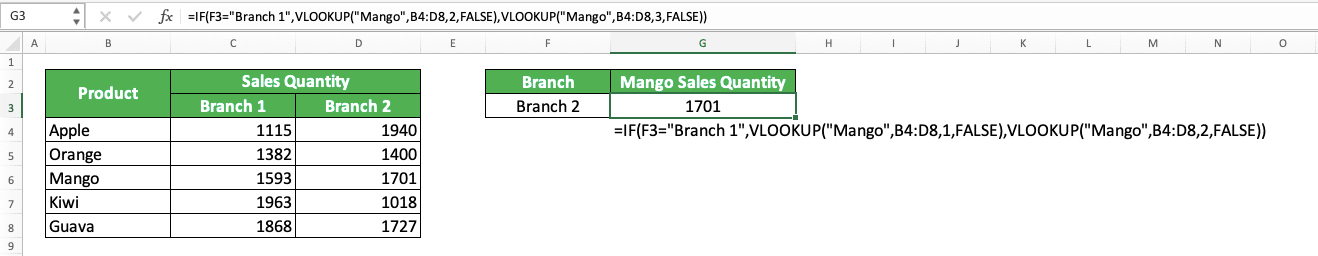IF VLOOKUP: Functions, Examples, and How to Use It in Excel - Compute Expert

# IF VLOOKUP: Functions, Examples, and How to Use It in Excel

Home >> Excel Tutorials from Compute Expert >> Excel Formulas List >> IF VLOOKUP: Functions, Examples, and How to Use It in Excel

In this tutorial, you will learn how to combine IF and VLOOKUP functions properly in excel.

When working in excel, we might sometimes need to get our data lookup result based on a particular value. If we know how to combine IF and VLOOKUP functions in excel, then we might be able to do that easier.

Want to know more about IF VLOOKUP and master the way to use them in one formula writing in excel? Read this tutorial until its last part!

Disclaimer: This post may contain affiliate links from which we earn commission from qualifying purchases/actions at no additional cost for you. Learn more

## How to Use IF VLOOKUP 1: Find Data for a Particular Value

When looking up data in excel using VLOOKUP, we might sometimes want to be dynamic depending on a cell value.

If the cell value is this, then we want to VLOOKUP like this. If the cell value is that, then we want to VLOOKUP like that. For this kind of situation, we can combine IF and VLOOKUP to help us get the result we want.

Here is the general writing form of the IF and VLOOKUP combination for this purpose.

= IF ( cell = value , VLOOKUP ( lookup_value1 , reference_range1 , result_column_index1 , [ lookup_mode1 ] ) , VLOOKUP ( lookup_value2 , reference_range2 , result_column_index2 , [ lookup_mode2 ] )

By combining IF and VLOOKUP like this, we can do our VLOOKUP based on our cell value. We can differentiate the VLOOKUP inputs and get different results depending on whether the cell contains our preferred value or not.

To better understand the concept, here is its implementation example in excel.As you can see in the example, we have a reference table with one sales quantity column for each branch. There are two branches here and we might want to find our sales quantity data from either one of them.

If we combine IF and VLOOKUP, then we can determine from which branch we get the number of our sales easily. Just input a cell containing the branch from which we want to get the number of our sales to our IF. Next, input a VLOOKUP that looks up for the branch’s sales quantity and a VLOOKUP that looks up for another branch.

By doing that, we can just change our cell value to another branch to get its sales quantity. Here is what happens if we change the branch cell value in the example to “Branch 2”.If you need more than two VLOOKUP alternatives, just nest your IFs according to the number of VLOOKUPs you need.

## How to Use IF VLOOKUP 2: Produce a Result for a Particular Data Find

Another function of the IF and VLOOKUP combination is to produce a result from a particular VLOOKUP data find.

If you need this function, you just need to write the VLOOKUP in the logic condition input part of the IF. Compares it with the data find you expect from the VLOOKUP.

If the comparison is correct, then the IF will produce its TRUE result. If it isn’t, then the IF will produce its FALSE result.

Here is the general writing form of the IF and VLOOKUP combination for this purpose.

= IF ( VLOOKUP ( lookup_value , reference_range , result_column_index , [ lookup_mode ] ) = data , result_if_true , result_if_false )

And here is its implementation example in excel.In the example, we want to mark the test score we find with “pass” or “fail”. If the test score is equal to or more than 80, then we mark it with “pass”. If it is lower than 80, then we mark it with “fail”.

Thus, we use VLOOKUP to find the test score inside the IF and compares its result with 80. As the logic condition we input there is equal to or more than 80, the IF true result is “pass”. On the other hand, input “fail” as the IF false result.

By combining the IF and VLOOKUP like that, we immediately get the correct mark for Patrick’s test score in the example!

## Exercise

After you have learned how to use IF VLOOKUP in excel completely, now let’s do an exercise. This is so you can understand the lessons from this tutorial more practically.

### Questions

Combine IF and VLOOKUP to get your answers! Answer in the appropriate gray-colored cell according to the question number.
1. How many inventories does apple have in region B?
2. How many inventories does apple have in region A? Use the same formula writing as the one you use in question no. 1 except for the region cell!
3. If the inventory quantity is less than 750, then we should mark it as “Needs More Stock”. Otherwise, we should mark it as “OK”.

What is the mark for the inventory quantity of guava in region B?

If you have excel 2019 or a later excel version, you can also combine your VLOOKUP with IFS instead of IF. This combination might be better for you, especially if you have more than two VLOOKUP alternatives to consider.

Related tutorials you should learn from:

Get updated excel info from Compute Expert by registering your email. It's free!